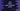# Java program to check if a matrix is upper triangular matrix or not### Java program to check if a matrix is upper triangular matrix or not :

In this tutorial, we will learn how to find if a matrix is upper triangular or not. A matrix is called upper triangular if all the elements of the matrix below the main diagonal is 0. Else, it is not an upper triangular matrix.

Our Java program will first take the inputs for the matrix from the user and then it will check if it is upper triangular or not. For example, below matrix is upper triangular :

``````1        2        3
0        4        5
0        0        6``````

The main diagonal is 1-4-6 and all elements are 0 below the diagonal.

### How to solve this problem :

Let?s take one example, below matrix is an upper triangular matrix :

``````1        2        3        4
0        5        6        7
0        0        8        9
0        0        0        10``````

Let?s try to analyze it :

1. row = 0 , total 0 = 0
2. row = 1 , total 0 = 1, for column 0
3. row = 2 , total 0 = 2, from column 0 to column 1
4. row = 3 , total 0 = 3, from column 0 to column 2

So, for line no. i, we will check for all column positions starting from 0 to i - 1. If all are 0, it is not an upper triangular matrix. Else, it is . Let?s do this programmatically :

### Java program to check upper triangular matrix :

``````import java.util.Scanner;

class Main {
public static void main(String args[]) {
//1
int row, col;
boolean isUpperTriangular = true;

//2
Scanner scanner = new Scanner(System.in);
System.out.println("Enter total number of rows : ");
row = scanner.nextInt();

//3
System.out.println("Enter total number of columns : ");
col = scanner.nextInt();

//4
int inputArray[][] = new int[row][col];

//5
for (int i = 0; i < row; i++) {
for (int j = 0; j < col; j++) {
System.out.println("Enter element for array[" + (i + 1) + "," + (j + 1) + "] : ");
inputArray[i][j] = scanner.nextInt();
}
}

//6
System.out.println("You have entered : ");
for (int i = 0; i < row; i++) {
for (int j = 0; j < col; j++) {
System.out.print(inputArray[i][j] + "\t");
}
System.out.println();
}

//7
for (int i = 0; i < row; i++) {
for (int j = 0; j < i; j++) {
if (inputArray[i][j] != 0) {
isUpperTriangular = false;
break;
}
}
if (!isUpperTriangular) {
break;
}
}

//8
if(isUpperTriangular){
System.out.println("Upper triangular matrix.");
}else{
System.out.println("Not an Upper triangular matrix.");
}
}
}``````

### Explanation :

1. Create two integers row and col to store the row and columns for the matrix.isUpperTriangular value is a flag to determine if it is a upper triangular or not. If yes, it?s value will be true , else false.
2. Create one scanner object to read the user input values. Ask the user to enter the rows count. Read and store it in row variable.
3. Similarly, read the total count of columns for the matrix and store it in col variable.
4. Create one two dimensional integer array inputArray. The row and columns of this array is save as the user given row and col values.
5. Run two for loops and read all elements for the matrix. Read and store them in the two dimensional array.
6. Print out the matrix to the user. Since we are storing it in a two dimensional array, add one new line after each row.
7. Now, scan all elements of the matrix using two loops. The outer loop will run from i = 0 to i = row -1. Inner loop will run from j = 0 to j = i -1. That means, it will check only the values below the main diagonal of the matrix. Check for each item if it is 0 or not. If not, set the value of isUpperTriangular = false and break from both loop. One break will break from the inner loop. We are checking again using an if and breaking from the outer loop.
8. Finally, based on the flag value, print if it is a upper triangular matrix or not.

### Sample Output :

``````Enter total number of rows :
3
Enter total number of columns :
3
Enter element for array[1,1] :
1
Enter element for array[1,2] :
2
Enter element for array[1,3] :
3
Enter element for array[2,1] :
0
Enter element for array[2,2] :
4
Enter element for array[2,3] :
5
Enter element for array[3,1] :
0
Enter element for array[3,2] :
0
Enter element for array[3,3] :
6
You have entered :
1        2        3
0        4        5
0        0        6
-> Upper triangular matrix.

Enter total number of rows :
3
Enter total number of columns :
3
Enter element for array[1,1] :
1
Enter element for array[1,2] :
2
Enter element for array[1,3] :
3
Enter element for array[2,1] :
4
Enter element for array[2,2] :
5
Enter element for array[2,3] :
6
Enter element for array[3,1] :
7
Enter element for array[3,2] :
8
Enter element for array[3,3] :
9
You have entered :
1        2        3
4        5        6
7        8        9
-> Not an Upper triangular matrix.``````